Courses

# Test: Factorisation

## 10 Questions MCQ Test HJ TEST bundle data | Test: Factorisation

Description
This mock test of Test: Factorisation for Class 9 helps you for every Class 9 entrance exam. This contains 10 Multiple Choice Questions for Class 9 Test: Factorisation (mcq) to study with solutions a complete question bank. The solved questions answers in this Test: Factorisation quiz give you a good mix of easy questions and tough questions. Class 9 students definitely take this Test: Factorisation exercise for a better result in the exam. You can find other Test: Factorisation extra questions, long questions & short questions for Class 9 on EduRev as well by searching above.
QUESTION: 1

Solution:
QUESTION: 2

### Factoring 3x2-5x+2

Solution:

3x^2-3x-2x+2
now, we take common
3x(x-1)-2(x-1)
(x-1)common and (3x-2)
(x-1)(3x-2)

QUESTION: 3

### The number of zeros of x2 + 4x + 2

Solution:
QUESTION: 4

Factorise by splitting the middle term 2x- 11x + 12

Solution:
QUESTION: 5

The volume of cuboid is given by the expression x3+2x2-x-2. The dimension of the cuboid for x = 5 is:​

Solution:
QUESTION: 6

Expansion of 2x(x + 2y) + 3x(2x - 3y) yields

Solution:
QUESTION: 7

What are the two factors of quadratic polynomial x2-16x+64?

Solution: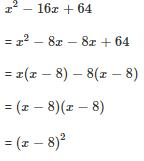QUESTION: 8

Factorise the quadratic polynomial by splitting the middle term:
x2 + 14x + 45

Solution:

The two numbers that add up to 14, and multiply to 45 and 9 and 5.

(x+5)(x+9)

QUESTION: 9

Factorize the quadratic polynomial by splitting the middle term: y2 – 4 y –21​

Solution:
QUESTION: 10

What is the value of p if x-2 is a factor of x2 – 6x + p ?

Solution:

P(x) = ×2-6×+p
p (2)= (2)2-6 (2) +p
4- 12 + p
-8 + p
p = -8

Track your progress, build streaks, highlight & save important lessons and more!

### Similar Content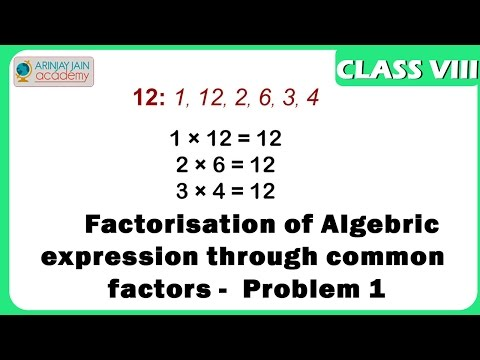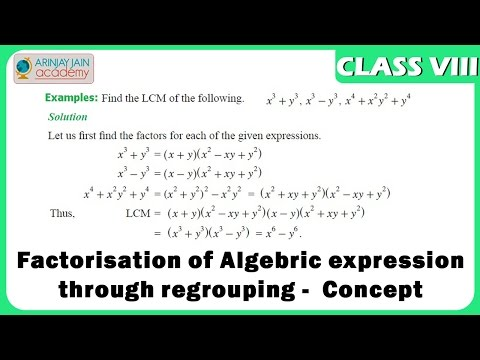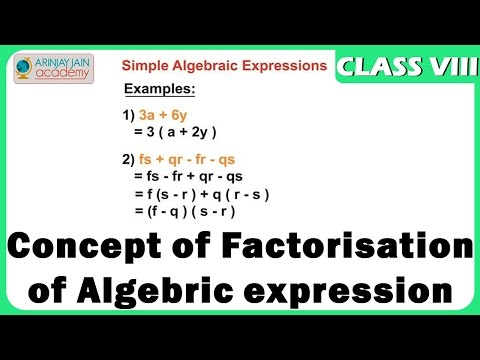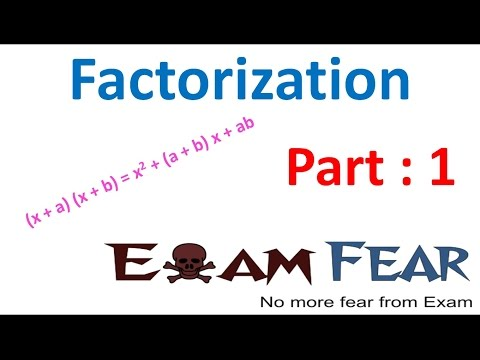### Related tests